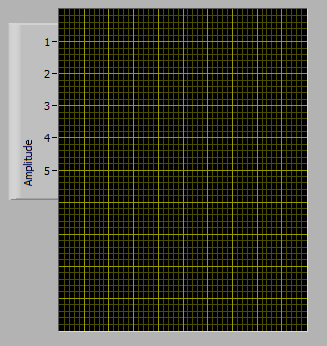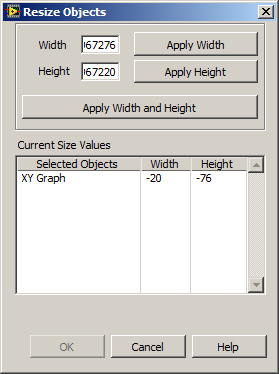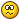## Recommended Posts

A few times now I've needed to dynamically display and resize some XY graphs. And it never seems to fail that after a few iterations I end up with a chart that resembles this:Or worse, I end up with a chart that has a negative size according to LabVIEW:Once this happens there is no way I have found to manually resize the graph. I have to delete the control and drop a new one, and in the process lose my formatting, local variables, property nodes, event cases, etc. "Replace" gives a new control, but at the exact same (negative) size.

Today I was fed up and looked to why this happens. This is what I learned.

First, you need to know there are two main movable parts to the graph. The first is the whole graph control. This works as you'd expect using the "Position" property. The second is the "plot area" and it is affected by "Plot Area Bounds" and "Plot Area:Size". From the development environment the of size the plot area is constrained by the Graph Control -- you can't make the plot area bigger than the control. When resizing programmatically, the plot area can be sized/moved anywhere in the pane that owns the graph control.

Second, properties "Plot Area Bounds" and "Plot Area:Size" do similar things, but affect the Graph Control differently. "Plot Area:Size" will change the size of the plot area, and it will change the size of the Graph Control. If you use this property to increase the width of the plot area by 10 pixels, the width of the Graph Control will increase by 10 pixels. If you use this property to decrease the width of the plot area by 25 pixels, the width of the Graph Control will decrease by 25 pixels. This will be important later.

"Plot Area Bounds" will change the size and position of the plot area without doing anything to the Graph Control. If you forget "Plot Area Bounds" is referenced to the owning pane and not the Graph Control, its easy to have your plot area moved to upper left corner of the window while the rest of your graph control sits in the bottom middle.

The WTF. If you use "Plot Area Bounds" to make the size of the plot area bigger than the Graph Control, say 100 pixels wider, you can now use "Plot Area:Size" to shrink the plot area to 75 pixels wide and get a Graph Control that is -25 pixels wide.Simple Move\Resize:

This is the typical use case, you need to resize the graph and you don't care how the plot area is aligned within it.

1. Move the graph to the desired position using the "Position" property.
2. Resize the plot area using "Plot Area:Size" and the graph control will resize by the same amount.

Resize Graph and resize plot area:

In my case I need to align the plot areas of multiple graphs. Because the Y Axis scales are formatted differently, the plot areas of each graph have slightly different positions.

1. Write data to the graph
2. Update the scales
3. Move the graph to the desired position using the "Position" property.
4. Resize the Graph Control indirectly by using "Plot Area:Size" knowing this won't be the final position of the plot area.
5. Reposition the plot area using "Plot Area Bounds" recalling its parameters are referenced to the owning pane and not the graph control.

Attached is a VI (LV 2009) that'll demonstrate "Plot Area Bounds" and "Plot Area:Size"

DynamicGraphSizing.vi

•1

## Join the conversation

You can post now and register later. If you have an account, sign in now to post with your account.×   Pasted as rich text.   Paste as plain text instead

Only 75 emoji are allowed.

×   Your previous content has been restored.   Clear editor

×   You cannot paste images directly. Upload or insert images from URL.

×

×